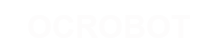#### Site Tools

en:reference:language:if

# Differences

This shows you the differences between two versions of the page.

 — en:reference:language:if [2017/04/07 10:03] (current) 2014/11/07 12:47 弘毅 created 2014/11/07 12:47 弘毅 created Line 1: Line 1: + =====if (conditional) and ==, !=, <, > (comparison operators)===== + + if, which is used in conjunction with a comparison operator, tests whether a certain condition has been reached, such as an input being above a certain number. The format for an if test is: + + + if (someVariable > 50) + { + // do something here + } + ​ + + The program tests to see if someVariable is greater than 50. If it is, the program takes a particular action. Put another way, if the statement in parentheses is true, the statements inside the brackets are run. If not, the program skips over the code. + The brackets may be omitted after an if statement. If this is done, the next line (defined by the semicolon) becomes the only conditional statement. + + + if (x > 120) digitalWrite(LEDpin,​ HIGH); ​ + + if (x > 120) + digitalWrite(LEDpin,​ HIGH); ​ + + if (x > 120){ digitalWrite(LEDpin,​ HIGH); } + + if (x > 120){ + digitalWrite(LEDpin1,​ HIGH); + digitalWrite(LEDpin2,​ HIGH); ​ + }                                 // all are correct + ​ + + The statements being evaluated inside the parentheses require the use of one or more operators: + + === Comparison Operators: === + + <​code>​ + x == y (x is equal to y) + x != y (x is not equal to y) + x <  y (x is less than y)  ​ + x >  y (x is greater than y) + x <= y (x is less than or equal to y) + x >= y (x is greater than or equal to y) + ​ + + === Warning: === + + Beware of accidentally using the single equal sign (e.g. if (x = 10) ). The single equal sign is the assignment operator, and sets x to 10 (puts the value 10 into the variable x). Instead use the double equal sign (e.g. if (x == 10) ), which is the comparison operator, and tests whether x is equal to 10 or not. The latter statement is only true if x equals 10, but the former statement will always be true. + + This is because C evaluates the statement if (x=10) as follows: 10 is assigned to x (remember that the single equal sign is the assignment operator), so x now contains 10. Then the '​if'​ conditional evaluates 10, which always evaluates to TRUE, since any non-zero number evaluates to TRUE. Consequently,​ if (x = 10) will always evaluate to TRUE, which is not the desired result when using an '​if'​ statement. Additionally,​ the variable x will be set to 10, which is also not a desired action. + + if can also be part of a branching control structure using the [[reference:​language:​else|if...else]] construction. +